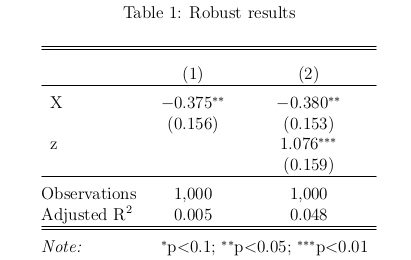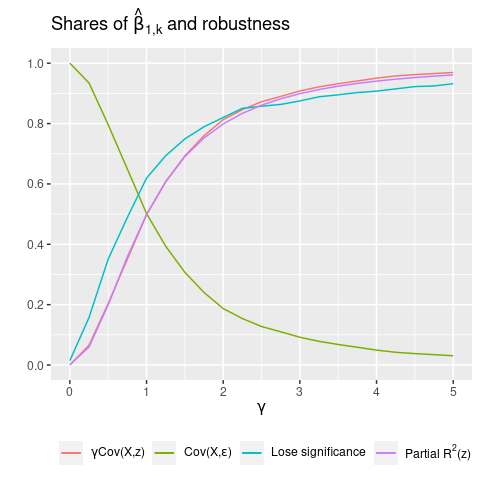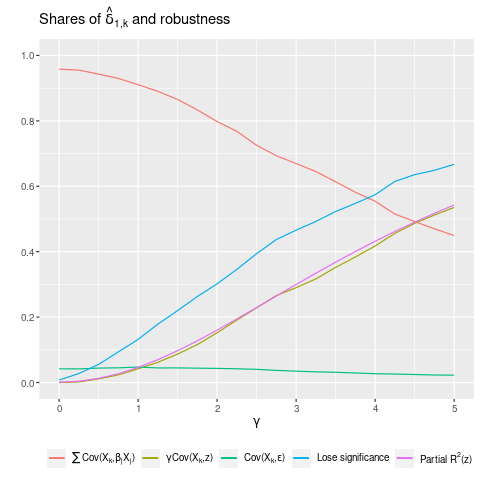January 16, 2021

# How to p-hack a robust result

Economists want to show that our results are robust, like in Table 1 below: Column 1 contains the baseline model, with no covariates, and Column 2 controls for $$z$$. Because the coefficient on $$X$$ is stable and significant across columns, we say that our result is robust.The twist: I p-hacked this result, using data where the true effect of $$X$$ is zero.

In this post, I show that it can be easy to p-hack a robust result like this. Here’s the basic idea: first, p-hack a significant result by running regressions with many different treatment variables, where the true treatment effects are all zero. For 20 regressions, we expect to get one false positive: a result with $$p<0.05$$. Then, using this significant treatment variable, run a second regression including a control variable, to see whether the result is robust to controls.

It turns out that the key to p-hacking robust results is to use control variables that have a low partial-$$R^{2}$$. These variables don’t have much influence on our main coefficient when excluded from the regression, and also have little influence when included. In contrast, controls with high partial-$$R^{2}$$ are more likely to kill a false positive. Lesson: high partial-$$R^{2}$$ controls are an effective robustness check against false positives.

# Setup

Let’s see how this works. Consider data for $$i=1, ..., N$$ observations generated according to

$\tag{1} y_{i} = \sum_{k=1}^{K} \beta_{k} X_{k,i} + \gamma z_{i} + \varepsilon_{i}.$

We have $$K$$ potential treatment variables, $$X_{1,i}$$ to $$X_{K,i}$$, and a control variable $$z_{i}$$. I draw $$X_{k,i} \sim N(0,1)$$, $$z_{i} \sim N(0,1)$$, and $$\varepsilon_{i} \sim N(0,1)$$, so that $$X_{k,i}$$, $$z_{i}$$, and $$\varepsilon_{i}$$ are all independent, but could be correlated in the sample. I set $$\beta_{k}=0$$ for all $$k$$, so that $$X_{k}$$ has no effect on $$y$$, and the true model is

$\tag{2} y_{i} = \gamma z_{i} + \varepsilon_{i}.$

I’m going to p-hack using the $$X_{k}$$’s, running $$K$$ regressions and selecting the $$k^{*}$$ with the smallest p-value. I p-hack the baseline regression of $$y$$ on $$X_{k}$$, by running $$K$$ regressions of the form

$\tag{3} y_{i} = \alpha_{1,k} + \beta_{1,k}X_{k,i} + \nu_{i}. % kinda abusing notation here, since beta_{1,k} is not the coefficient in the DGP (beta_{k})$

I use the ‘1’ subscript to indicate that this is the baseline model in Column 1. Out of these $$K$$ regressions, I select the $$k^{*}$$ with the smallest p-value on $$\beta_{1}$$. That is, I select the regression

$\tag{4} y_{i} = \alpha_{1,k^{*}} + \beta_{1,k^{*}}X_{k^{*},i} + \nu_{i}.$

When $$K\geq 20$$, we expect $$\hat{\beta}_{1,k^{*}}$$ to have $$p<0.05$$, since with a 5% significance level (i.e., false positive rate), the average number of significant results is $$20\times0.05 = 1$$. This is our p-hacked false positive.

To get a robust sequence of regressions, I need my full model including $$z$$ to also have a significant coefficient on $$X_{k^{*},i}$$. To test this, I run my Column 2 regression:

$\tag{5} y_{i} = \alpha_{2,k^{*}} + \beta_{2,k^{*}}X_{k^{*},i} + \gamma z_{i} + \varepsilon_{i}.$

Given that we p-hacked a significant $$\hat{\beta}_{1,k^{*}}$$, will $$\hat{\beta}_{2,k^{*}}$$ also be significant?

# Homogeneous $$\beta=0$$

First, I show a case where p-hacked results are not robust. I use the data-generating process from above with $$\beta=0$$.

When regressing $$y$$ on $$X_{k}$$ in the p-hacking step, we have

$\tag{6} y_{i} = \alpha_{1,k} + \beta_{1,k} X_{k,i} + \nu_{i},$

where

\tag{7} \begin{align} \nu_{i} &= \sum_{j \neq k}^{K} \beta_{1,j} X_{j,i} + \gamma z_{i} + \varepsilon_{i} \\ &= \gamma z_{i} + \varepsilon_{i}. \end{align}

We estimate the slope coefficient as

$\tag{8} \hat{\beta}_{1,k} = \frac{\widehat{Cov}(X_{k},y)}{\widehat{Var}(X_{k})} = \frac{\gamma \widehat{Cov}(X_{k},z) + \widehat{Cov}(X_{k},\varepsilon)}{\widehat{Var}(X_{k})}.$

Since $$\beta=0$$, we should only find a significant $$\hat{\beta}_{1,k}$$ due to a correlation between $$X_{k}$$ and the components of the error term $$\nu_{i}$$: (1) $$\gamma \widehat{Cov}(X_{k},z)$$, and (2) $$\widehat{Cov}(X_{k},\varepsilon)$$.

When $$\gamma \widehat{Cov}(X_{k},z)$$ is the primary driver of $$\hat{\beta}_{1,k}$$, controlling for $$z$$ in Column 2 will kill the false positive.

Turning to the full regression in Column 2, we get

\begin{align} \tag{9} \hat{\beta}_{2,k} &= \frac{\widehat{Cov}(\hat{u},y)}{\widehat{Var}(\hat{u})} = \frac{\widehat{Cov}((X_{k} - \hat{\lambda}_{1} z),\varepsilon)}{\widehat{Var}(\hat{u})} \\ &= \frac{\widehat{Cov}(X_{k},\varepsilon) - \hat{\lambda}_{1} \widehat{Cov}(z,\varepsilon)}{\widehat{Var}(\hat{u})}. \end{align}

This is from the two-step Frisch-Waugh-Lovell method, where we first regress $$X_{k}$$ on $$z$$ ($$X_{k} = \lambda_{0} + \lambda_{1} z + u$$) and take the residual $$\hat{u} = X_{k} - \hat{\lambda}_{0} - \hat{\lambda}_{1} z$$. Then we regress $$y$$ on $$\hat{u}$$, using the variation in $$X_{k}$$ that’s not due to $$z$$, and the resulting slope coefficient is $$\hat{\beta}_{2,k}$$.1 We can see that controlling for $$z$$ literally removes the $$\gamma \widehat{Cov}(X_{k},z)$$ term from our estimate.

Hence, to p-hack robust results, we want $$\hat{\beta}_{1,k}$$ to be driven by $$\widehat{Cov}(X_{k},\varepsilon)$$, since that term is also in $$\hat{\beta}_{2,k}$$. If we have a significant result that’s not driven by $$z$$, then controlling for $$z$$ won’t affect our significance.

## Simulations

Setting $$K=20, N=1000$$, and $$\gamma=1$$, I perform $$1000$$ replications of the above procedure: I run 20 regressions, select the most significant $$X_{k^{*}}$$ and record the p-value on $$\hat{\beta}_{1,k^{*}}$$, then add $$z$$ to the regression and record the p-value on $$\hat{\beta}_{2,k^{*}}$$. As expected when using a $$5\%$$ significance level, I find that out of the $$K$$ regressions in the p-hacking step, the average number of significant results is $$0.05$$. I find that $$\hat{\beta}_{1,k^{*}}$$ is significant in 663 simulations (=66%). But only 245 simulations (=25%) have both a significant $$\hat{\beta}_{1,k^{*}}$$ and a significant $$\hat{\beta}_{2,k^{*}}$$, meaning that only 37% (=245/663) of p-hacked Column 1 results have a significant Column 2. So in the $$\beta=0$$ case, we infer that $$\widehat{Cov}(X_{k},\varepsilon)$$ is small relative to $$\gamma \widehat{Cov}(X_{k},z)$$. With these parameters, it’s not easy to p-hack robust results.Figure 1 repeats this process for a range of $$\gamma$$’s. I plot the shares of $$\gamma \widehat{Cov}(X_{k},z)$$ and $$\widehat{Cov}(X_{k},\varepsilon)$$ in $$\hat{\beta}_{1,k}$$.2 We see that when $$\gamma=0$$, $$\gamma \widehat{Cov}(X_{k},z)$$ has 0 weight, but its share increases quickly. Closely correlated with this share is the fraction of significant results losing significance after controlling for $$z$$. Specifically, this is the fraction of simulations with a nonsignificant $$\hat{\beta}_{2,k}$$, out of the simulations with a significant $$\hat{\beta}_{1,k}$$. And even more tightly correlated with $$\gamma \widehat{Cov}(X_{k},z)$$ is the partial $$R^{2}$$ of $$z$$.3 Intuitively, as $$\gamma$$ increases, the additional improvement in model fit from adding $$z$$ also increases, which by definition increases $$R^{2}(z)$$. Hence, $$R^{2}(z)$$ turns out to be a useful proxy for the share of $$\gamma \widehat{Cov}(X_{k,i},z_{i})$$, which we can’t calculate in practice. Lesson: when partial-$$R^{2}(z)$$ is large, controlling for $$z$$ is an effective robustness check for false positives. This is because a large $$\gamma \widehat{Cov}(X_{k},z)$$ implies both (1) a large $$R^{2}(z)$$; and (2) that $$z$$ is more likely to be the source of the false positive, and hence controlling for $$z$$ will kill it. So now we have a new justification for including control variables, apart from addressing confounders: to rule out false positives driven by coincidental sample correlations.

# Heterogeneous $$\beta_{i} \sim N(0,1)$$

However, you might think that $$\beta=0$$ is not a realistic assumption. As Gelman says: “anything that plausibly could have an effect will not have an effect that is exactly zero.” So let’s consider the case of heterogeneous $$\beta_{i}$$, where each individual $$i$$ has their own effect drawn from $$N(0,1)$$. For large $$N$$, the average effect of $$X$$ on $$y$$ will be 0, but this effect will vary by individual. This is a more plausible assumption than $$\beta$$ being uniformly 0 for everyone. And as we’ll see, this also helps for p-hacking, by increasing the variance of the error term.

Here we have data generated according to

$\tag{10} y_{i} = \sum_{k=1}^{K} \beta_{k,i} X_{k,i} + \gamma z_{i} + \varepsilon_{i},$

where $$\beta_{k,i} \sim N(0,1)$$.

Then, when regressing $$y$$ on $$X_{k}$$, we have

$\tag{11} y_{i} = \alpha_{1,k} + \delta_{1,k} X_{k,i} + v_{i},$

where

$\tag{12} v_{i} = -\delta_{1,k} X_{k,i} + \beta_{k,i} X_{k,i} + \sum_{j \neq k}^{K} \beta_{j,i} X_{j,i} + \gamma z + \varepsilon_{i}.$

When effects are heterogeneous (i.e., we have $$\beta_{k,i}$$ varying with $$i$$), a regression model with a constant slope $$\delta_{1,k}$$ is misspecified. To emphasize this, I include $$-\delta_{1,k} X_{k,i}$$ in the error term.4

The estimated slope coefficient is

\tag{13} \begin{align} \hat{\delta}_{1,k} &= \frac{\widehat{Cov}(X_{k,i},y_{i})}{\widehat{Var}(X_{k,i})} \\ &= \frac{\sum_{j=1}^{K} \widehat{Cov}(X_{k,i},\beta_{j,i}X_{j,i}) + \gamma \widehat{Cov}(X_{k,i},z_{i}) + \widehat{Cov}(X_{k,i},\varepsilon)_{i}}{\widehat{Var}(X_{k,i})} \end{align}

From Aronow and Samii (2015), we know that the slope coefficient converges to a weighted average of the $$\beta_{k,i}$$’s:

$\tag{14} \hat{\delta}_{1,k} \rightarrow \frac{E[w_{i} \beta_{k,i}]}{E[w_{i}]},$

where $$w_{i}$$ are the regression weights: the residuals from regressing $$X_{k}$$ on the other controls. In this case, as we’re using a univariate regression, the residuals are simply demeaned $$X_{k}$$ (when regressing $$X$$ on a constant, the fitted value is $$\bar{X}$$).

Because $$\beta_{k,i} \sim N(0,1)$$, we have $$E[w_{i} \beta_{k,i}]=0$$ and hence $$\hat{\delta}_{1,k}$$ converges to 0. So any statistically significant $$\hat{\delta}_{1,k}$$ that we estimate will be a false positive.

There are three terms that make up $$\hat{\delta}_{1,k}$$ and could drive a false positive: (1) $$\sum_{j=1}^{K} \widehat{Cov}(X_{k,i},\beta_{j,i}X_{j,i})$$, (2) $$\gamma \widehat{Cov}(X_{k},z)$$, and (3) $$\widehat{Cov}(X_{k},\varepsilon)$$.

Now we have a new source of false positives, case (1), due to heterogeneity in $$\beta_{k,i}$$. Note that controlling for $$z$$ will only affect one out of three possible drivers, so now we should expect our false positives to be more robust to control variables, compared to when $$\beta=0$$. To see this, note that when controlling for $$z$$ in the full regression, we have

\tag{15} \begin{align} \hat{\delta}_{2,k} &= \frac{\widehat{Cov}(\hat{u}_{i},y_{i})}{\widehat{Var}(\hat{u}_{i})} \\ &= \frac{\sum_{j=1}^{K} \widehat{Cov}(X_{k,i} - \hat{\lambda}_{1} z_{i},\beta_{j,i}X_{j,i}) + \widehat{Cov}(X_{k,i} - \hat{\lambda}_{1} z_{i},\varepsilon_{i})}{\widehat{Var}(\hat{u}_{i})} \\ &= \frac{\sum_{j=1}^{K} \widehat{Cov}(X_{k,i},\beta_{j,i}X_{j,i}) + \widehat{Cov}(X_{k,i},\varepsilon_{i})}{\widehat{Var}(\hat{u_{i}})} \\ &- \hat{\lambda}_{1} \frac{\left[ \sum_{j=1}^{K} \widehat{Cov}(z_{i},\beta_{j,i}X_{j,i}) + \widehat{Cov}(z_{i},\varepsilon_{i}) \right] }{\widehat{Var}(\hat{u_{i}})} \end{align}

Here $$\hat{u}$$ is the residual from a regression of $$X_{k}$$ on $$z$$: $$X_{k} = \lambda_{0} + \lambda_{1} z + u$$. We obtain $$\hat{\delta}_{2,k}$$ by regressing $$y$$ on $$\hat{u}$$, via FWL, and using the variation in $$X_{k}$$ that’s not due to $$z$$.

Comparing $$\hat{\delta}_{1,k}$$ to $$\hat{\delta}_{2,k}$$, we see that $$\sum_{j=1}^{K} \widehat{Cov}(X_{k,i},\beta_{j,i}X_{j,i}) + \widehat{Cov}(X_{k_{i}},\varepsilon_{i})$$ shows up in both estimates. Hence, if our p-hacking selects for a $$\hat{\delta}_{1,k}$$ with a large value of these terms, we’re also selecting for the majority of the components of $$\hat{\delta}_{2,k}$$. In contrast to the $$\beta=0$$ case, now we should expect $$\gamma \widehat{Cov}(X_{k,i},z_{i})$$ to be dominated, and significance in Column 1 should carry over to Column 2.

## Simulations

I repeat the same procedure as before, running $$K=20$$ regressions of $$y$$ on $$X_{k}$$ and $$z$$, taking the $$X_{k}$$ with the smallest p-value, $$X_{k^{*}}$$, and then running another regression while excluding $$z$$. Again, I use $$\gamma=1$$ and perform $$1000$$ replications. Here I use robust standard errors to address heteroskedasticity.

I find that $$\hat{\delta}_{1,k^{*}}$$ is significant in 650 simulations (=65%). But this time, 569 simulations (=57%) have both a significant $$\hat{\delta}_{1,k^{*}}$$ and a significant $$\hat{\delta}_{2,k^{*}}$$. So 88% (=569/650) of p-hacked Column 1 estimates also have a significant Column 2. Compare this to 37% in the $$\beta=0$$ case. That’s what I call p-hacking a robust result! We infer that $$\gamma \widehat{Cov}(X_{k,i},z_{i})$$ is too small relative to the other components for its presence or absence to affect our estimates very much.

To illustrate how $$\hat{\delta}_{1,k}$$ is determined, I plot the shares of its three constituent terms while varying $$\gamma$$.5As shown in Figure 2, when $$\gamma$$ is small, most of the weight in $$\hat{\delta}_{1,k}$$ is from $$\sum_{j=1}^{K} \widehat{Cov}(X_{k,i},\beta_{j,i}X_{j,i})$$, indicating that its $$K$$ terms provide ample opportunity for correlations with $$X_{k^{*},i}$$. But as $$\gamma$$ increases, this share falls, while the share of $$\gamma \widehat{Cov}(X_{k,i},z_{i})$$ rises linearly. The share of $$\widehat{Cov}(X_{k,i},\varepsilon_{i})$$ is small and decreases slightly. Looking at robustness, we see that the fraction of significant results losing significance rises much more slowly than in the $$\beta=0$$ case. And we again see a tight link between partial-$$R^{2}(z)$$ and the share of $$z$$ in $$\hat{\delta}_{1,k}$$.6

Overall, we can see why controlling for $$z$$ is less effective with heterogeneous effects: $$\hat{\delta}_{1,k}$$ is mostly not determined by $$\gamma \widehat{Cov}(X_{k,i},z_{i})$$, so removing it (by controlling for $$z$$) has little effect. In other words, when variables have low partial-$$R^{2}$$, controlling for them won’t affect false positives.

# Conclusion

In general, economists think about robustness in terms of addressing potential confounders. I haven’t seen any discussion of robustness to false positives based on coincidental sample correlations. This is possibly because it seems hopeless: we always have a 5% false positive rate, after all. But as I’ve shown, adding high partial-$$R^{2}$$ controls is an effective robustness check against p-hacked false positives.7 So we have a new weapon to combat false positives: checking whether a result remains significant as high partial-$$R^{2}$$ controls are added to the model.

## Footnotes

1. $$\widehat{Cov}(\hat{u},y) = \widehat{Cov}(\hat{u},\gamma z + \varepsilon) = \gamma \widehat{Cov}(\hat{u},z) + \widehat{Cov}(\hat{u},\varepsilon) = 0 + \widehat{Cov}(\hat{u},\varepsilon)$$, since the residual $$\hat{u}$$ is orthogonal to $$z$$.

2. Note that these terms can be negative, so this is not strictly a share in $$[0,1]$$. When the terms in the denominator almost cancel out to 0, we get extreme values. Hence, for each $$\gamma$$, I take the median share across all simulations, which is well-behaved.

3. $$R^{2}(z) = \frac{\sum \hat{u}_{i}^{2} - \sum \hat{v}_{i}^{2}}{\sum \hat{u}_{i}^{2}}$$, where $$\hat{u}_{i}^{2}$$ is the residual from the baseline model, and $$\hat{v}_{i}^{2}$$ is the residual from the full regression (where we control for $$z$$). In other words, partial $$R^{2}(z)$$ is the proportional reduction in the sum of squared residuals from adding $$z$$ to the model. See also the R function and Wikipedia

4. We could write $$\beta_{k,i} = \bar{\beta}_{k,i} + (\beta_{k,i}-\bar{\beta}_{k,i}) := b_{k} + b_{k,i}$$, and then have $$y_{i} = \alpha_{1,k} + b_{k} X_{k,i} + v_{i}$$, with $$v_{i} = b_{k,i}X_{k,i} + \sum_{j \neq k}^{K} \beta_{j,i} X_{j,i} + \gamma z_{i} + \varepsilon_{i}$$. However, $$\hat{b}_{k}$$ does not generally converge to $$b_{k} = \bar{\beta}_{k,i}$$, as I discuss below.

5. Similar results hold when varying $$Var(\beta_{i})$$ or $$Var(\varepsilon)$$.

6. Note that the overall $$R^{2}$$ in Column 1 is irrelevant. For $$\alpha=0.05$$, we will always have a false positive rate of 5% when the null hypothesis is true. Controlling for $$z$$ is effective when $$\gamma \widehat{Cov}(X_{k,i},z_{i})$$ has a large share in $$\hat{\delta}_{1,k}$$. And a large share also means that $$R^{2}(z)$$ is large. This is true whether the overall $$R^{2}$$ is 0.01 or 0.99, since partial $$R^{2}$$ is defined in relative terms, as the decrease in the sum of squared residuals relative to a baseline model.

7. Note that this holds regardless of which regression is p-hacked. Here, I’ve p-hacked the baseline regression. But the results are actually identical when you work backwards, p-hacking the full regression and then excluding $$z$$.